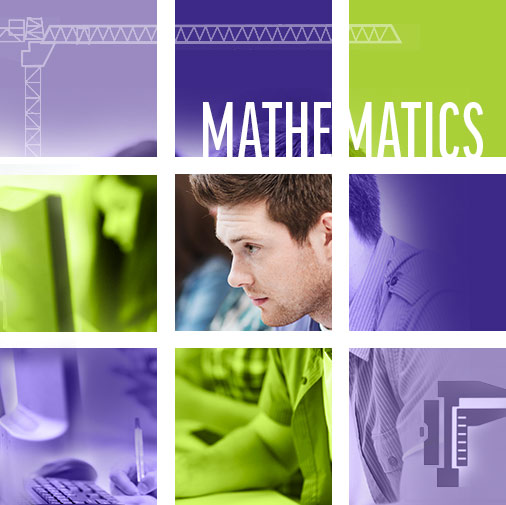# Pre-Calculus 11

Mark Swiednicki

## Prerequisite

Foundations and Pre-Calculus Mathematics 10
or Principle of Math 10
or LF Math 7: Math Foundations

## Description

This Math 11 course is aimed at students who plan on completing post-secondary programs that require the knowledge of theoretical calculus, such as sciences or business.

Students use headphones or speakers to hear narration and explanation while the material is presented online. Students have complete control over the pace at which they learn the lesson—lessons can be paused, resumed, and repeated using simple navigation buttons. Examples are interactive with animated step-by-step flash and video whiteboard solutions. The assignments and tests however are done by hand and submitted either electronically or by mail.

online

4

Student paced

## Evaluation

20% of the school mark comes from the send-in assignments

80% of the school mark comes from the tests

BC Performance Standards

## Support

SIDES teacher to provide face to face, telephone, e-mail or online support.

## Materials

Students need a scientific calculator

UNIT ONE: ABSOLUTE VALUE AND RADICALS

Lesson 1 - Real Numbers and Absolute Value
Lesson 4 - Multiplying, Dividing and Rationalizing Radicals
Lesson 5 - Solving Radical Equations

UNIT TWO: FACTORING PLYNOMIALS

Lesson 1 - Common Factors
Lesson 2 - Factoring Simple Trinomials
Lesson 3 - Factoring Messy Trinomials
Lesson 4 - Difference of Squares and Perfect Trinomial Squares
Lesson 5 - Factoring by Substitution and Grouping

UNIT THREE: RATIONAL EXPRESSIONS

Lesson 1 - Comparing Rational Expressions to Rational Numbers
Lesson 2 - Non-Permissible Values for Rational Expressions
Lesson 3 - Simplifying Rational Expressions
Lesson 4 - Multiplying and Dividing Rational Expressions
Lesson 5 - Adding and Subtracting Rational Expressions
Lesson 6 - Solving Problems Involving Rational Expressions

Lesson 1 - Quadratic Functions - Translations
Lesson 2 - Quadratic Functions Expansions, Compressions
Lesson 3 - Determining Equations of Quadratic Functions
Lesson 4 - Changing from General Form to Standard Form
Lesson 5 - Graphing Linear and Quadratic Reciprocal Functions

Lesson 1 - Solving Quadratics by Factoring
Lesson 2 - Solving Quadratics by the Square Root Principle
Lesson 4 - The Discriminant

UNIT SIX: SOLVING SYSTEMS OF EQUATIONS

Lesson 1 - Solving Quadratic Inequalities
Lesson 2 - Graphing Linear Inequalities in Two Variables
Lesson 3 - Solving Linear-Quadratic Systems in Two Variables
Lesson 5 - Solving Linear-Quadratic Inequalities in Two Variables

UNIT SEVEN: ARITHMETIC AND GEOMETRIC SEQUENCES AND SERIES

Lesson 1 - Arithmetic Sequences
Lesson 2 - Arithmetic Series
Lesson 3 - Geometric Sequences
Lesson 4 - Geometric Series
Lesson 5 - Infinite Geometric Series

UNIT EIGHT: TRIGONOMETRY

Lesson 1 - Angles in Standard Position
Lesson 2 - Trig Ratios of Angles in Standard Position
Lesson 3 - Exact Values Sine, Cosine, and Tangent of Special Angles
Lesson 4 - Solving Conditional Trig Equations
Lesson 5 - Deriving the Sine Law
Lesson 6 - Application of the Sine Law
Lesson 7 - Sine Law and the Ambiguous Case
Lesson 8 - Deriving the Cosine Law
Lesson 9 - Application of the Cosine Law

UNIT NINE: ABSOLUTE VALUE & RECIPROCAL FUNCTIONS

Lesson 1: Absolute Value Functions
Lesson 2: Solving Absolute Value Equations
Lesson 3: Graphing Linear Reciprocal Functions
Lesson 4: Graphing Reciprocal Quadratic Functions

## Outcomes